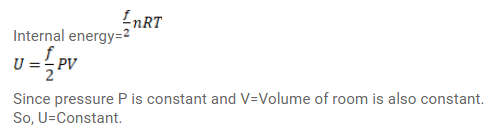# Use R=8.3 J/mol-K wherever required. Show that the internal energy of the air (treated as an ideal gas)Question:

Use $\mathrm{R}=8.3 \mathrm{~J} / \mathrm{mol}-\mathrm{K}$ wherever required.

Show that the internal energy of the air (treated as an ideal gas) contained in a room remains constant as the temperature changes between day and night. Assume that the atmospheric pressure around remains constant and the air in the room maintains this pressure by communicating with the surrounding through the windows etc.

Solution: